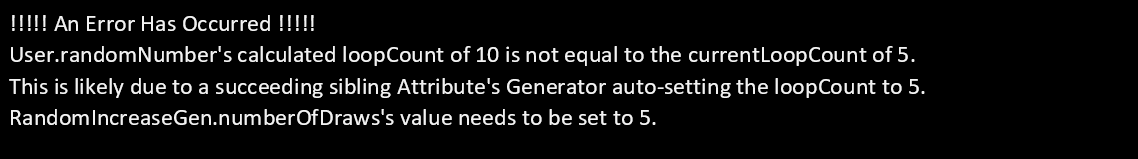# Description

The RandomIncDecPeakGen Generator can be used to generate a random increasing and decreasing sequence of numbers (whole and decimal), where the position of the "peak" (highest) number in the sequence can be defined. The total of the numbers will be the "targetsum." (ex: give me 5 numbers totaling 35, with the sequence peak in position 3).

## When should RandomIncDecPeakGen be used?

• Any time a user wants to generate a random, finite set of numbers with a defined slope (i.e., peak) that totals a specific value.

• The peak of the slope can be defined at any point within the defined number of draws and will be the highest point in the generated finite sequence.

## When should RandomIncDecPeakGen not be used?

• Any time a user wants to generate a random set of numbers in no particular order where the total is irrelevant.

• Whenever a user wants to generate patterned data where the generated numbers cannot just be any random number.

## Generator Parameters

The following parameters may be configured for the RandomIncDecPeakGen Generator. Items with an asterisk* are required.

• targetSum* - Defines the target amount that all values must add up to based on the number of draws. The targetSum can be a positive or negative value.
• numberOfDraws* - Defines the finite number of values to be randomly generated in increasing order and must equal the sum defined in the targetSum parameter. The number of draws parameter value must be greater than '0'.
• peak* - Defines the draw index to peak on and is limited to the maximum number of draws defined within the numberOfDraws parameter. This means depending on the peak chosen, the slope before the peak can go from shallow to deep or vice versa on the slope after the peak. If the peak is zero (default), a mid-peak will be determined, and both sides of the peak will have very similar slopes.
• format* - Defines the format of a value when it is decimal instead of an integer. Examples: #0.##, #0.00, #0
• seed - Defines an optional seed. Using a seed ensures the same random sequence is repeated each time test data is generated.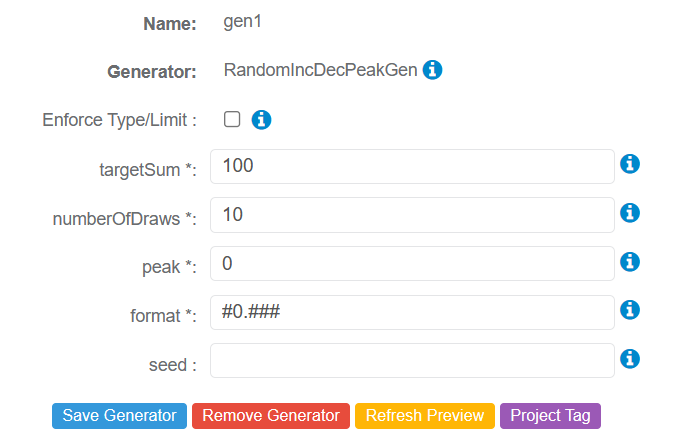## numberOfDraws Parameter and Domain loopCount

• The numberOfDraws parameter sets the Domain loopCount.

• If the Domain loopCount exceeds the numberOfDraws parameter value, record generation will stop at whatever value is entered as the number of draws within the RandomIncDecPeakGen Generator.

• Example - If the Domain loopCount is '20 "and the numberOfDraws parameter is '10', then only 10 records will be generated. The values generated for those 10 records will equal the targetSum parameter value.numberOFDraws = 10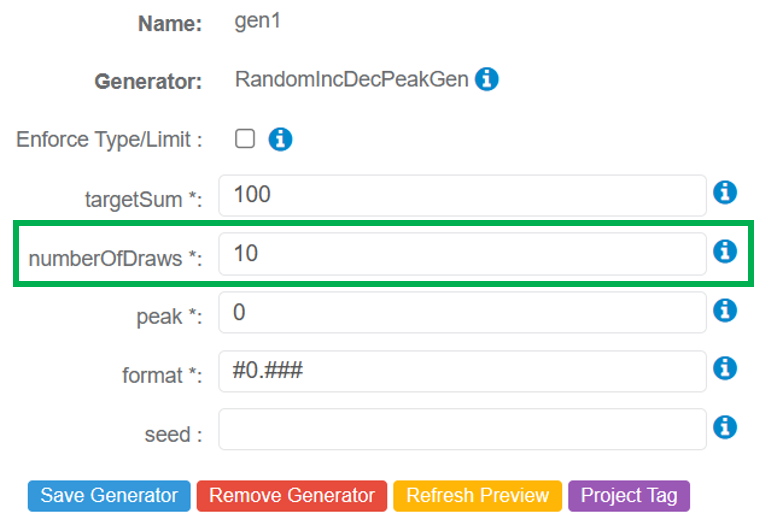The result is 10 records that total 100.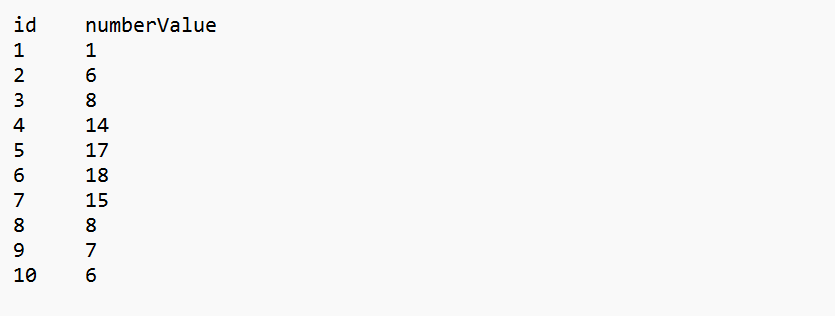## Example 1 - Generate 10 Values, Peaking in the Middle, Totaling 100

This example uses the Generator's default values. The peak of the slope will be in the middle, and the slope will be similar on both sides.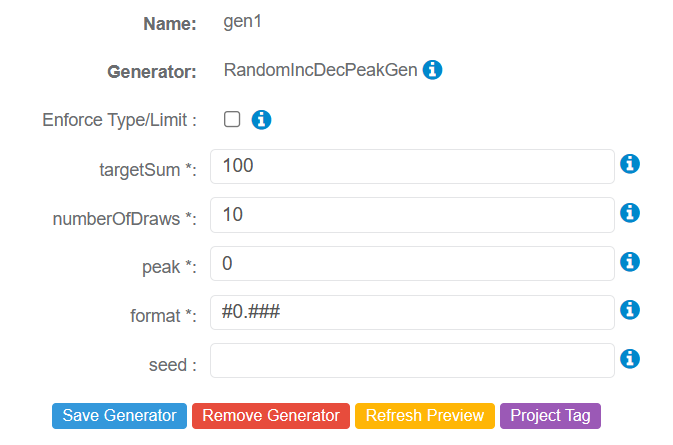Sample Output

For this example, the peak value is position #6. The values together total '100'.## Example 2 - Generate 10 Values, Peaking at Position 8, Totaling 100

This example shows how to generate values where the peak of the slope occurs later in the number sequence.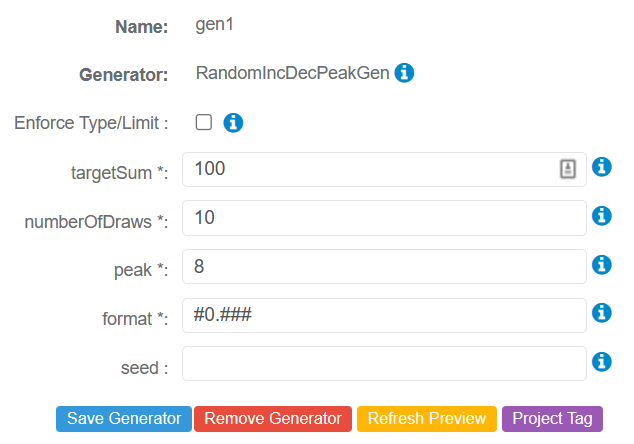Sample Output

In this example, the peak value is in position # 8. The numbers will increase through position 8 and then decrease until the defined number of draws is reached.## Example 3 - Generate 8 Values, Peaking at Position 3, that Total 50

This example shows how to generate values where the peak of the slope occurs earlier in the number sequence.Sample Output

In this example, the resulting output will be decimal values. This is because 8 does not go evenly into 50.

(50/8 = 6.25)## Special Use Case - Using RandomIncDecPeakGen with RandomIncreaseGen

The numberOfDraws parameter within the RandomIncDecPeakGen and RandomIncreaseGen Generators must be the same value when assigned to one or more Attributes within the same Domain.

Example of Improper Configuration
In the example below, the Domain has two Attributes:

1. randomNumber - assigned RandomIncreasGen
2. randomNumber2 - assigned RandomIncDecPeakGen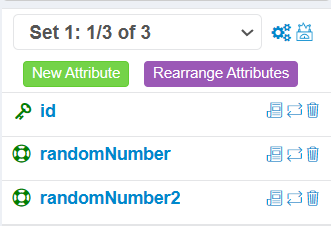The RandomIncreaseGen Generator has been assigned to the randomNumber Attribute. The numberOfDraws parameter has a value of '10".The RandomIncDecPeakGen Generator has been assigned to the randomNumber2 Attribute. The numberOfDraws parameter has a value of '5'. The randomNumber2 Attribute's Generator will be used to set the loopCount.Since the values differ for the numberOfDraws parameter, the following error will be received when generating test data with GenRocket Runtime.

To fix the error, the value must be the same for the numberOfDraws parameter in both Generators (RandomIncDecPeakGen and RandomIncreaseGen).